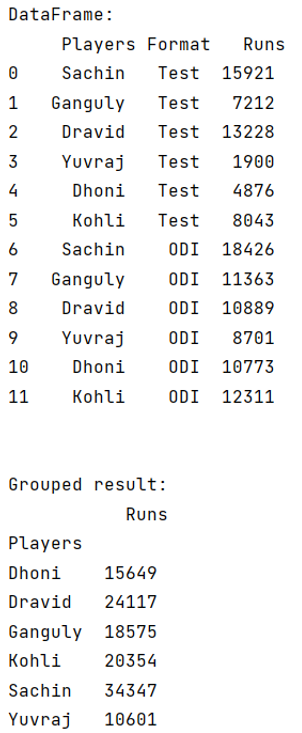# Python Pandas groupby sort within groups

Learn about the groupby sort within groups in Python Pandas. By Pranit Sharma Last updated : September 20, 2023

## Problem statement

Given a Pandas DataFrame, we have to groupby sort within groups in Python Pandas.

## Pandas groupby sort within groups

For groupby sort within groups in Python Pandas, we will use the df.groupby() method by specifying the column name and sort=True parameter and then use the .sum() method. The groupby() method is a simple but very useful concept in pandas. By using groupby(), we can create a grouping of certain values and perform some operations on those values.

The groupby() function split the object, apply some operations, and then combines them to create a group hence a large amount of data and computations can be performed on these groups. Sorting within groups is nothing but arranging the result of groupby() in ascending or descending order.

Syntax:

```DataFrame.groupby(
by=None,
axis=0,
level=None,
as_index=True,
sort=True,
group_keys=True,
squeeze=NoDefault.no_default,
observed=False,
dropna=True
)
```
Note

To work with pandas, we need to import pandas package first, below is the syntax:

```import pandas as pd
```

Let us understand with the help of an example.

## Python program for Pandas groupby sort within groups

```# Importing pandas package
import pandas as pd

# creating a dictionary of student marks
d={
"Players":['Sachin','Ganguly','Dravid','Yuvraj','Dhoni','Kohli',
'Sachin','Ganguly','Dravid','Yuvraj','Dhoni','Kohli'],
"Format":['Test','Test','Test','Test','Test','Test',
'ODI','ODI','ODI','ODI','ODI','ODI'],
"Runs":[15921,7212,13228,1900,4876,8043,
18426,11363,10889,8701,10773,12311]
}

# Now we will create DataFrame
df = pd.DataFrame(d)

# Viewing the DataFrame
print("DataFrame:\n",df,"\n\n")

# Performing sum on groupby on Players with runs
result = df.groupby(['Players'], sort=True).sum()

# Display Result
print("Grouped result:\n",result)
```

### Output

The output of the above program is:We can observe in the above example, by passing sort = True, all the names are sorted alphabetically.